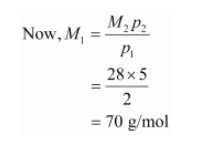# At 0°C, the density of a certain oxide of a gas at 2 bar is same as that of dinitrogen at 5 bar.

Question:

At 0°C, the density of a certain oxide of a gas at 2 bar is same as that of dinitrogen at 5 bar. What is the molecular mass of the oxide?

Solution:

Density (d) of the substance at temperature (T) can be given by the expression,

$d=\frac{M p}{R T}$

Now, density of oxide (d1) is given by,

$d_{1}=\frac{M_{1} p_{1}}{\mathrm{R} T}$

Where, M1 and p1 are the mass and pressure of the oxide respectively.

Density of dinitrogen gas (d2) is given by,

$d_{2}=\frac{M_{2} p_{2}}{R T}$

Where, M2 and p2 are the mass and pressure of the oxide respectively.

According to the given question,

$d_{1}=d_{2}$

$\therefore M_{1} p_{1}=M_{2} p_{2}$

Given,

$p_{1}=2 b a r$

$p_{2}=5 \mathrm{bar}$

Molecular mass of nitrogen, $M_{2}=28 \mathrm{~g} / \mathrm{mol}$Hence, the molecular mass of the oxide is 70 g/mol.